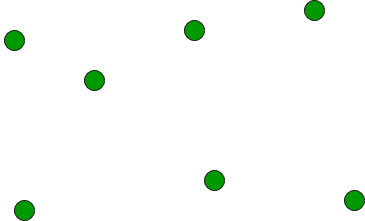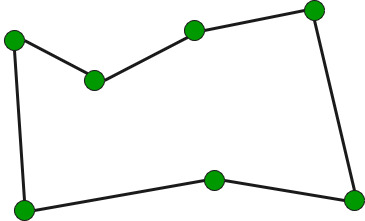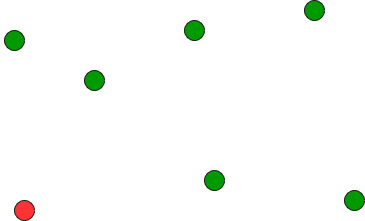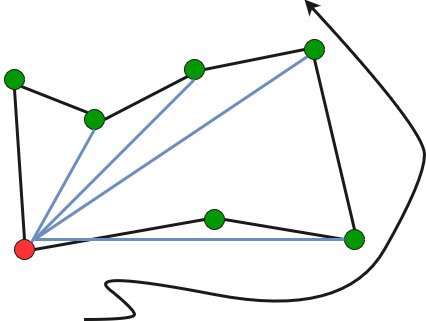# Find Simple Closed Path for a given set of points

• Difficulty Level : Hard
• Last Updated : 13 Jul, 2022

Given a set of points, connect the dots without crossing.Example:

```Input: points[] = {(0, 3), (1, 1), (2, 2), (4, 4),
(0, 0), (1, 2), (3, 1}, {3, 3}};

Output: Connecting points in following order would
not cause any crossing
{(0, 0), (3, 1), (1, 1), (2, 2), (3, 3),
(4, 4), (1, 2), (0, 3)}```

We strongly recommend you to minimize your browser and try this yourself first.
The idea is to use sorting.

• Find the bottom-most point by comparing y coordinate of all points. If there are two points with same y value, then the point with smaller x coordinate value is considered. Put the bottom-most point at first position.• Consider the remaining n-1 points and sort them by polor angle in counterclockwise order around points. If polor angle of two points is same, then put the nearest point first.
• Traversing the sorted array (sorted in increasing order of angle) yields simple closed path.How to compute angles?
One solution is to use trigonometric functions.
Observation: We don’t care about the actual values of the angles. We just want to sort by angle.
Idea: Use the orientation to compare angles without actually computing them!

Below is C++ implementation of above idea.

## C++

 `// A C++ program to find simple closed path for n points``// for explanation of orientation()``#include ``using` `namespace` `std;` `struct` `Point``{``    ``int` `x, y;``};` `// A global point needed for  sorting points with reference``// to the first point. Used in compare function of qsort()``Point p0;` `// A utility function to swap two points``int` `swap(Point &p1, Point &p2)``{``    ``Point temp = p1;``    ``p1 = p2;``    ``p2 = temp;``}` `// A utility function to return square of distance between``// p1 and p2``int` `dist(Point p1, Point p2)``{``    ``return` `(p1.x - p2.x)*(p1.x - p2.x) +``           ``(p1.y - p2.y)*(p1.y - p2.y);``}` `// To find orientation of ordered triplet (p, q, r).``// The function returns following values``// 0 --> p, q and r are collinear``// 1 --> Clockwise``// 2 --> Counterclockwise``int` `orientation(Point p, Point q, Point r)``{``    ``int` `val = (q.y - p.y) * (r.x - q.x) -``              ``(q.x - p.x) * (r.y - q.y);` `    ``if` `(val == 0) ``return` `0;  ``// collinear``    ``return` `(val > 0)? 1: 2; ``// clockwise or counterclock wise``}` `// A function used by library function qsort() to sort``//  an array of points with respect to the first point``int` `compare(``const` `void` `*vp1, ``const` `void` `*vp2)``{``   ``Point *p1 = (Point *)vp1;``   ``Point *p2 = (Point *)vp2;` `   ``// Find orientation``   ``int` `o = orientation(p0, *p1, *p2);``   ``if` `(o == 0)``     ``return` `(dist(p0, *p2) >= dist(p0, *p1))? -1 : 1;` `   ``return` `(o == 2)? -1: 1;``}` `// Prints simple closed path for a set of n points.``void` `printClosedPath(Point points[], ``int` `n)``{``   ``// Find the bottommost point``   ``int` `ymin = points.y, min = 0;``   ``for` `(``int` `i = 1; i < n; i++)``   ``{``     ``int` `y = points[i].y;` `     ``// Pick the bottom-most. In case of tie, chose the``     ``// left most point``     ``if` `((y < ymin) || (ymin == y &&``         ``points[i].x < points[min].x))``        ``ymin = points[i].y, min = i;``   ``}` `   ``// Place the bottom-most point at first position``   ``swap(points, points[min]);` `   ``// Sort n-1 points with respect to the first point.``   ``// A point p1 comes before p2 in sorted output if p2``   ``// has larger polar angle (in counterclockwise``   ``// direction) than p1``   ``p0 = points;``   ``qsort``(&points, n-1, ``sizeof``(Point), compare);` `   ``// Now stack has the output points, print contents``   ``// of stack``   ``for` `(``int` `i=0; i

Output:

```(0, 0), (3, 1), (1, 1), (2, 2), (3, 3),
(4, 4), (1, 2), (0, 3), ```

Time complexity of above solution is O(n Log n) if we use a O(nLogn) sorting algorithm for sorting points.
Auxiliary Space: O(1), since no extra space has been taken.

Source:
http://www.dcs.gla.ac.uk/~pat/52233/slides/Geometry1x1.pdf